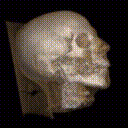## Fast Volume Rendering Using a Shear-Warp Factorization of the Viewing Transformation

Philippe Lacroute, Ph.D. dissertation, Technical Report CSL-TR-95-678, Stanford University, 1995.

### Abstract:

Volume rendering is a technique for visualizing 3D arrays of sampled data. It has applications in areas such as medical imaging and scientific visualization, but its use has been limited by its high computational expense. Early implementations of volume rendering used brute-force techniques that require on the order of 100 seconds to render typical data sets on a workstation. Algorithms with optimizations that exploit coherence in the data have reduced rendering times to the range of ten seconds but are still not fast enough for interactive visualization applications. In this thesis we present a family of volume rendering algorithms that reduces rendering times to one second.

First we present a scanline-order volume rendering algorithm that exploits coherence in both the volume data and the image. We show that scanline-order algorithms are fundamentally more efficient than commonly-used ray casting algorithms because the latter must perform analytic geometry calculations (e.g. intersecting rays with axis-aligned boxes). The new scanline-order algorithm simply streams through the volume and the image in storage order. We describe variants of the algorithm for both parallel and perspective projections and a multiprocessor implementation that achieves frame rates of over 10 Hz.

Second we present a solution to a limitation of existing volume rendering algorithms that use coherence accelerations: they require an expensive preprocessing step every time the volume is classified (i.e. when opacities are assigned to the samples), thereby limiting the usefulness of the algorithms for interactive applications. We introduce a data structure for encoding spatial coherence in unclassified volumes. When combined with our rendering algorithm this data structure allows us to build a fully-interactive volume visualization system.

Click on any chapter heading to retrieve a Postscript file containing the text of the chapter (with low-resolution grayscale images). The full text of the dissertation is also available.
• Front Matter (Postscript, 49 KBytes)
• Abstract
• Acknowledgements
• Contents
• List of Tables
• List of Figures
• Chapter 1: Introduction (Postscript, 280 KBytes)
• Volume Rendering
• The Volume Rendering Equation
• The Volumetric Compositing Approximation
• Data Representation and Sampling
• The Visualization Process
• A New Family of Fast Volume Rendering Algorithms
• Organization
• Chapter 2: Prior Work (Postscript, 53 KBytes)
• Volume Rendering Algorithms
• Ray Casting
• Splatting
• Cell Projection
• Multipass Resampling
• Acceleration Techniques
• Spatial Data Structures
• Early Ray Termination
• Chapter Summary
• Chapter 3: The Shear-Warp Factorization (Postscript, 427 KBytes)
• An Overview of the Factorization
• The Affine Factorization
• The Perspective Factorization
• Properties of the Factorization
• Existing Shear-Warp Algorithms
• Chapter Summary
• Chapter 4: Three Fast Volume Rendering Algorithms (Postscript, 632 KBytes)
• Parallel Projection Rendering Algorithm
• Overview of the Parallel Projection Algorithm
• The Run-Length Encoded Volume
• The Run-Length Encoded Image
• Resampling the Volume
• Warping the Intermediate Image
• Opacity Correction
• Implementation of the Parallel Projection Algorithm
• Perspective Projection Rendering Algorithm
• Overview of the Perspective Projection Algorithm
• Resampling the Volume
• Opacity Correction
• Implementation of the Perspective Projection Algorithm
• Limitations of the Perspective Projection Algorithm
• Fast Classification Algorithm
• Overview of the Fast Classification Algorithm
• The Min-Max Octree
• The Summed-Area Table
• Implementation of the Fast Classification Algorithm
• Limitations of the Fast Classification Algorithm
• Switching Between Modes
• Chapter Summary
• Chapter 5: Performance Analysis (Postscript, 1314 KBytes)
• Performance of the Shear-Warp Algorithms
• Speed and Memory Performance
• Image Quality
• Comparison of Coherence-Accelerated Volume Rendering Algorithms
• Asymptotic Complexity
• Experimental Methodology
• Comparison of Speedups from Algorithmic Optimizations
• Costs of Coherence Accelerations
• Analysis of the Shear-Warp Coherence Data Structures
• Low-Coherence Volumes
• Categories of Volume Data
• Voxel Throughput
• The Impact of Coherence on Rendering Time
• The Role of Coherence in Visualization
• Chapter Summary
• Chapter 6: A Multiprocessor Volume Rendering Algorithm (Postscript, 493 KBytes)
• Multiprocessor Rendering Algorithm
• Image and Object Partitions
• Data Distribution
• Overall Algorithm
• Implementation
• Hardware Architectures
• Software Implementation
• Results
• Rendering Rates
• Performance Limits on the Challenge Multiprocessor
• Performance Limits on the DASH Multiprocessor
• Memory Performance
• Related Work
• 6.4 Chapter Summary
• Chapter 7: Extensions (Postscript, 815 KBytes)
• Flexible Shading With Lookup Tables
• Shade Trees and Lookup Tables
• Fast Depth Cueing
• Depth Cueing
• Factoring the Depth Cueing Function
• Implementation of Fast Depth Cueing
• Implementation of the Shadow Rendering Algorithm
• Performance of the Shadow Rendering Algorithm
• Rendering Mixtures of Volume Data and Polygons
• Clipping Planes
• Chapter Summary
• Chapter 8: VolPack: A Volume Rendering Library (Postscript, 63 KBytes)
• System Architecture
• Volume Representation
• Viewing Model
• Functionality Provided by VolPack
• Chapter Summary
• Chapter 9: Conclusions (Postscript, 35 KBytes)
• Final Summary
• Future Directions for Performance Improvements
• Hardware Support for Volume Rendering
• Interactive Volume Rendering
• Appendix A: Mathematics of the Shear-Warp Factorization (Postscript, 400 KBytes)
• Coordinate Systems and Definitions
• The Affine Factorization
• Finding the Principle Viewing Axis
• Transformation to Standard Object Coordinates
• The Shear and Warp Factors
• Projection to the Intermediate Image
• The Complete Affine Factorization
• The Perspective Factorization
• Finding the Principle Viewing Axis
• Transformation to Standard Object Coordinates
• The Shear and Warp Factors
• Projection to the Intermediate Image
• The Complete Perspective Factorization
• Bibliography (Postscript, 43 KBytes)